# Calculus of Variations and Variational Algorithms

This article will consider the main problems in the calculus of variations. Also the application of variational algorithms in machine learning. Therefore, if you are ready to dive into one of the very interesting topics of mathematics and you are a fan of machine learning, keep reading.

At the end of the article there will be an explanation of the theory of probabilistic deep neural networks, in which . variational derivation

variational calculusis an area of ​​calculus that uses variations, which are small changes in functions and functionals, for finding the maxima and minima of functionals: mappings from a set of functions to real numbers. Functionals are often expressed as definite integrals involving functions and their derivatives.

This definition from Wikipedia can be reformulated as follows – we study changes in functions or functionals, look for extremums … Am I the only one who thinks that this part of mathematical analysis has sufficient similarities with the mathematics of such models as neural networks. After considering the theory of the Calculus of Variations, there will be a description of the answer to this question (spoiler I think you already know)

## Examples of problems in the calculus of variations

I propose to consider / repeat a couple of basic equations and concepts. I will write mathematical definitions, and describe them in a simpler language.

One of the most important definitions in this area is functional. Let there be a set of functions satisfying some conditions. law or ruleaccording to which each function from the given set fits perfectly certain numerical value, is called a functional defined on a given set of functions

If given a function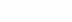then through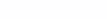denoted increment independent variable x.

And if we take a set of functions and consider them as integrals, we get the functional

But in functionality I[y(x)] argument increment y(x) is called a variation of the function y(x) and is denoted by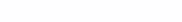The closer the curve y_1(x) to the curve y(x) , the less δy

Such a concept as functional increment I[y(x)] given in the form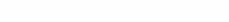Variation functionality — generalization of the concept of differential functions one variable, the main linear part of the increment functionality along a certain direction. The concept is used in the theory of extremal problems to obtain necessary and sufficient conditions for an extremum. It is this meaning that is put into this term, starting from the work of 1762 by J. Lagrange

Extremes

I remind you that finding extrema functions (minimums or maxima) is reduced to finding the point at which the derivative of the function is zero. Extremes functionals can be obtained by finding functions whose functional derivative is equal to zero. Which leads to the solution of the Euler-Lagrange equation

If the values ​​of the functional I[y(x)] on all curves sufficiently close to the curve y = y_0(x) less than I[y_0(x)] then we say that the functional I[y(x)] reaches its maximum on the curve y = y_0(x)

The functional is understood as an integral. In other wordsthe curve y_0(x) is the maximum for the functional I[y(x)] if all other values ​​of the functional lessthan the value of the functional from this curve(y_0(x)).

In this case, the inequality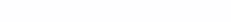If only on such a curve, the increment of the functional is zero, then it is said that on this curve the functional reaches absolute maximum.

The same story with the minimum, the curve y_0(x) is the minimum for the functional I[y(x)]if all other values ​​of the functional morethan the value of the functional from this curve(y_0(x)).

We have looked at the basic concepts when working with distributions of functions.

## Variational Algorithm in Machine Learning Model

When I talked about the similarity of variational problems and the theory of neural networks, I did not mean that the differential calculus is somehow similar to the variational calculus. After all, in the calculus of variations, functions are sought that deliver an extremum to the functionals. The assumption is the similarity tasksnot implementation.

It turns out that variational algorithms are used in some neural network models where the analytical solution is undecidable.

In neural algorithms (and not only), we are always looking for a point in the parameter space at which the loss function has a minimum value. The calculus of variations appears at the moment when we transform the loss function into another loss function, (often this is the lower bound on the variation), using the methods of the calculus of variations.

Variational methodsis a family methods approximations of intractable integrals arising from Bayesian inference .

For example, in Variational autoencoders, Expectation–maximization algorithm or in the implementation of Bayesian statistics in the neural network “Bayes by Backprop”

In order to make everything clear, let’s take an example – “Bayes by Backprop” in probabilistic neural networks.

## Variational Inference in the “Bayes by Backrop” Algorithm

In general, this architecture of the neural network is very interesting, below will be an explanation of the main concepts and, of course, the variational part

Here you need to be familiar with the basics of probability and Bayesian statistics.

First, we need to understand that we are representing weights as variance and mean in a distribution.

Here we define the approximate distribution that we want to optimize so that it is as close as possible to the unknown true distribution.

In the theory of such algorithms, under θ you need to understand the weights of the model, and by D the data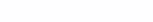In mathematics, an equation has already been defined for estimating the divergence between two distributions – KL – divergence

Naturally, we would need to define this as a loss function and minimize it, but…

We are faced with the problem of an unsolvable integral.

It is at this step that calculations with Variational Inference begin…

The solution to the problem is to apply the maximization of the following function:

I recommend that you read the CONCLUSION of this decision, (because this is the topic of the article)

Calculus of variations applied to this function

If you take a closer look at the KL discrepancy, you will notice that it is very similar to a checkmate! (E)

If we move to our optimization function and do some calculations, we get this difference:

In this article, it is MANDATORY to introduce the term Variational Lower Bound (ELBO).

Let’s say we have a function

The lower bound on this function will be any function

satisfying the equation:

here is an example of the bottom border

I think almost everyone guesses where the last formula is the lower limit